# Simplify

Simplify powers multiplication:
(3+22)(5-42)

x =  -77

### Step-by-step explanation: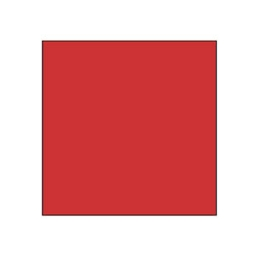Did you find an error or inaccuracy? Feel free to write us. Thank you!## Related math problems and questions:

• Simplify 7Simplify. 7-1/3÷ 3-2/3 of 2+ 4-1/2÷ 2-1/4+ 1/2 solution and by step by step
• RootThe root of the equation (x-19)2 -10 = x2 -11x is (equal or greater or less than zero)? ...
• Simplify:Simplify: 8(−9 − 5x) + 4b use x = 9 and b = −9
• Evaluate expressionEvaluate following expression: 5 to the power of 2 - (4x4) divided by 2 =
• Powers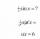Is true for any number a,b,c,d equality:? ?
• The schoolyardThe schoolyard had the shape of a square with an 11m side. The yard has been enlarged by 75 m2 and has a square shape again. How many meters was each side of the yard enlarged?
• Derivative problemThe sum of two numbers is 12. Find these numbers if: a) The sum of their third powers is minimal. b) The product of one with the cube of the other is maximal. c) Both are positive and the product of one with the other power of the other is maximal.
• 3x square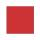The side length of the square is 54 cm. How many times increases the content area of a square if the length of side increase three times?
• GranddaughterIn 2014, the sum of the ages of Meghan's aunt, her daughter and her granddaughter was equal to 100 years. In what year was the granddaughter born, if we know that the age of each can be expressed as the power of two?
• Flowerbed 2Around the square flower bed in a park is sidewalk 2 m wide. The area of ​​this sidewalk is 243 m2. What is the area of the flowerbed?
• TilesHow many tiles of 20 cm and 30 cm can build a square if we have a maximum of 100 tiles?
• CircleHow big is an area of a circle if its circumference is 51.2 cm?
• Square Number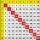If to a square of integer number add 17, we get the square of the next integer number. What is the original number?
• Trapezium zoomHow many times increase area of trapezoid if all sides and altitude increase 5 times?
• Simplest form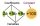Find the simplest form of the following expression: 3 to the 2nd power - 1/4 to the 2nd power.
• The cubeThe cube has a surface of 600 cm2. What is its volume?
• Evaluate 12Evaluate and simplify 9 divided by 7/6.